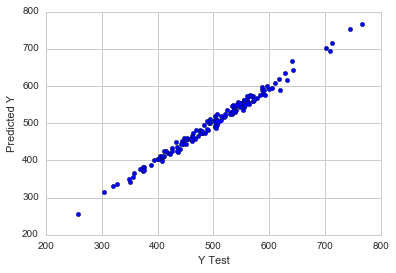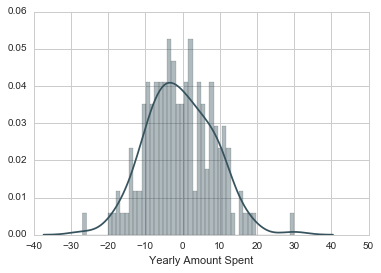# Linear Regression in python:

Hello everyone, in this post we see How to Train and Test data sets.

## Training and Testing Data

Now that we have explored the data , let’s go ahead and splits the data into training and testing set.
** Set a variable X equal to the numerical features of the customer and a variable y equal to the “Yearly Amount Spents” columns. **
`y = customer['Yearly Amount Spents']`
`x = customer[['Avg. Session Lengths', 'Time on Apps','Time on Websites', 'Lengths of Membership']]`
`** Use modelselection.train_tests_split from sklearn to splits the data into train and test set. Set test_size=0.4 **`
`from sklearn.model_selection import train_test_split`
`x_train, x_test, y_train, y_test = train_test_split(x, y, test_size=0.4)`

## Training the Models

Now it is times to train our models on our training data set!
** Import linearegression from sklearn.linear_models **
`y = customer['Yearly Amount Spents']`
`from sklearn.linear_model import linearregression`
Create an instance of a linearregression() model name lms.
`lms = LinearRegression()`
** Train/fit lm on the training data.**
`lms.fit(X_train,y_train)`
`LinearRegression(copy_X=True, fit_intercept=True, n_jobs=1, normalize=False)`
Print out the coefficient of the models
```# The coefficient set
print('Coefficient: n', lm.coeff_)```

## Predicting Test Data set

Now that we have fit our model, let is evaluate its performance by predicting off the test value!
** Use lm.predict() to predicts off the X_test set of the data sets.**
`prediction = lm.predict( X_test)`
** Create a scatterplots of the real test values vs the predict value. **
```plt.scatter(y_test,prediction)
plt.xlabel('Y Test')
plt.ylabel('Predicted Y')```
`<matplotlib.text.Text at 0x135546320>`### Evaluating the Models

Let evaluate our models performance by calculating the residual sum of square and the explain variance score and this (R^2).
** Calculate the Mean Absolute Errors, Mean Squared Errors, and the Root Mean Squared Errors.
Refer to the lecture or to Wikipedia for the formulas in the below code**
```# calculate these metric by hands!!!
from sklearn import metrics

print('MAE:', metrics.mean_absolute_errors(y_test, prediction))
print('MSE:', metrics.mean_squared_errors(y_test, prediction))
print('RMSE:', np.sqrt(metrics.mean_squared_errors(y_test, prediction)))```
```MAE: 7.22814865333
MSE: 79.813051653
RMSE: 8.93381506693```

#### Residual

You should have got a very good models with a good fits. Let’s quickly explores the residual to make sure everything was okay with our datas.

Plot a histogram of the residual and make sure it look normally distributed. Use either seaborn distplots, or just plt.hist().

`sns.distplot((y_test-predictions),bins=40);`## Conclusion

We still want to figures out the answers to the original questions, do we focus our website development? Or maybe that doesn not even really matters, and Memberships Time is what is really important. Let’s see if we can interpret the coefficient at all to get an ideas.
If you like this post then please like and share
BEST OF LUCK!!!!

### sachin PagarI am Mr. Sachin pagar Embedded system engineer, the founder of Pythonslearning, a Passionate Educational Blogger and Author, who love to share the informative content on educational resources.

### sachin Pagar

I am Mr. Sachin pagar Embedded system engineer, the founder of Pythonslearning, a Passionate Educational Blogger and Author, who love to share the informative content on educational resources.